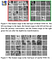# How to Ghost your Neural Network

## Insights from Han et. Al: “GhostNet: More Features from Cheap Operations”

For image classification & object detection, “GhostNet” yields similar or better performance 33% to 46% faster than state-of-the-art nets.

Imagine you’ve got a math problem that involves finding a number of different answers to several equations. Han’s team found a way to calculate a few of the answers, then duplicate & alter those answers to solve the rest of the problem in half the time. They did this using (in their own words) ghosts.

# Seeing Double

In case you aren’t too sure how a CNN works, don’t worry, most of us aren’t either. The basic idea is that you run an image through convolutional layers, which run filters with different sizes (3x3, 7x7 pixels etc) and algorithms over the pixels in the image to get an ‘average’ idea of what each pixel sort of looks like. This helps your model recognize “features” in the image — it allows your net to generalize.
For example, in a cat-or-not-cat image classification net, convolutional layers can start to recognize tails, ears, eyes and such, even in different shapes and angles.32 maps using convolution+ghosting vs. convolution. Red and green’s corresponding maps look fairly similar even to the naked eye

After establishing that there’s plenty of redundancy in normal CNNs, the team designed a “ghost module”: an alternative convolutional layer that runs linear transformations on fewer convoluted feature maps.

`Calculating the number of Floating Point OPerations for a layer:Input data: X ∈ R^(c*h*w)   c = number of input channels  h, w = height, width of input imageOutput data: Y = X [conv] f+b = convolutional layer generating n feature maps  [conv] = convolution operation  b = bias  Y ∈ R^(h'*w'*n) = output feature map with n channels    h', w' = height, width of output image  f ∈ R^(c*k*k*n) = the layer's convolution filters    k*k = size of convolutional filters. 3x3 etc    c = number of channelsNumber of FLOPs in layer = n*h'*w'*c*k*k = num_filters * output_size * num_channels * kernel_size`

# Testing Spooky Models

The publicly-available benchmark CIFAR-10, Imagenet ILSVRC 2012 and MS COCO datasets were fed into various architectures to judge performance. The team measured their own “GhostNet” and haunted versions of the state-of-the-art VGG-16, ResNet-50, and MobileNetV3 networks against the specter-free originals.

Written by